## Excel DB Function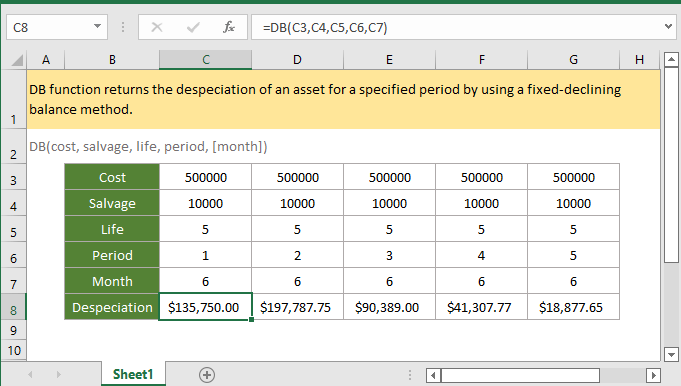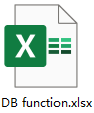### Description

The DB function returns the depreciation of an asset for a specified period by using a fixed-declining balance method.

### Syntax and arguments

Formula syntax

DB(cost, salvage, life, period, [month])

Arguments

 Cost: Required, the initial cost of the asset. Salvage: Required, the value of the asset at the end of the depreciation, or called the salvage value of the asset. Life: Required, the number of periods over which the asset will be depreciation, or the useful life of the asset. Period: Required, the period which used to calculate the depreciation for. Month: Optional, the number of months in the first year which is used in the calculation of the first period of depreciation. If it is omitted, the function takes the default value of 12.

Return Value

The DB function returns a numeric value in currency format.

Errors

1. If the arguments are non-numeric value, the function returns #VALUE! error value.

2. If anyone of below occurs, the function returns ##NUM! error value:

• Cost < 0, or salvage < 0;
• Life or period <= 0;
• Month <=0, or month > 12;
• Period > life;

Remarks

1. Both of the life and period must be in the same unit. For instance, the life is calculated in yearly, the period must be calculated in yearly too.

2. The fixed-declining balance method computes the depreciation of asset at a fixed rate. The formula used in DB function to calculate the depreciation for a period is:

=(Cost – Total depreciation from prior periods) * Rate

Where

Rate = 1 – ((salvage / cost) ^ (1 / life)).

However, different formulas are used for first and last period (first year, and the end of the depreciation).

For the first period, DB function uses the below formula:

=Cost * Rate * Month / 12

For the last period, DB function uses the below formula:

=((Cost – Total depreciation from prior periods) * Rate * (12 – month)) / 12

Version

Excel 2003 or later

### Usage and Examples

Example 1 when argument month is omitted

Supposing the cost, salvage, life and periods (1-5) are listed in the table B3:G8 and months are omitted, to calculate the depreciation of the asset in each period, please use below formula:

=DB(C3,C4,C5,C6)

Press Enter key to get the depreciation of first period.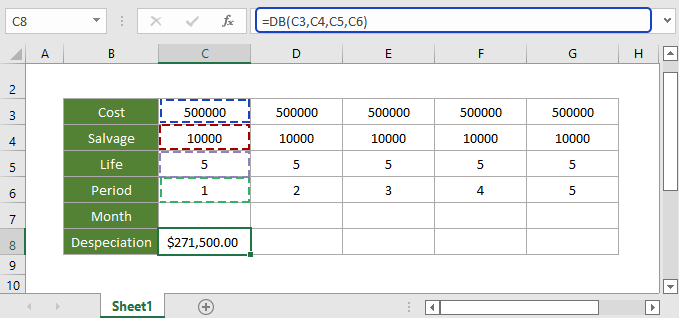Then select first result, drag autofill handle over the cells to calculate the depreciations in other periods.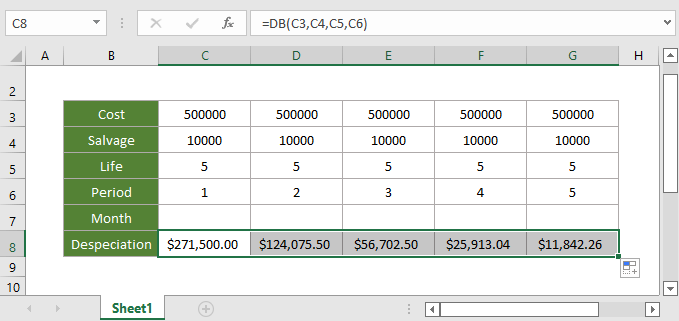Example 2 when argument month is less than 12

Supposing in the table B3:G8 which lists the cost, salvage, life and periods (1-5), and the month is 6, to calculate the depreciation of the asset in each period, please use below formula:

=DB(C3,C4,C5,C6,C7)

Press Enter key to get the depreciation of first period.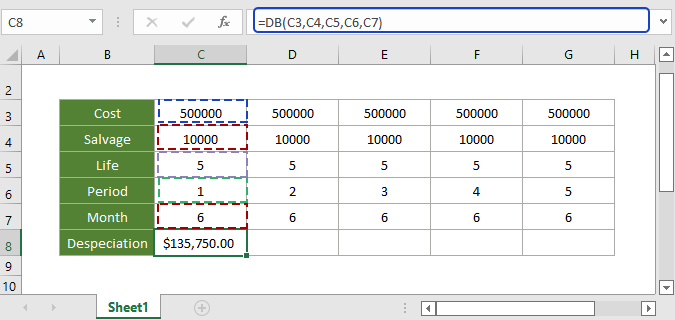Then select first result, drag autofill handle over the cells to calculate the depreciations in other periods.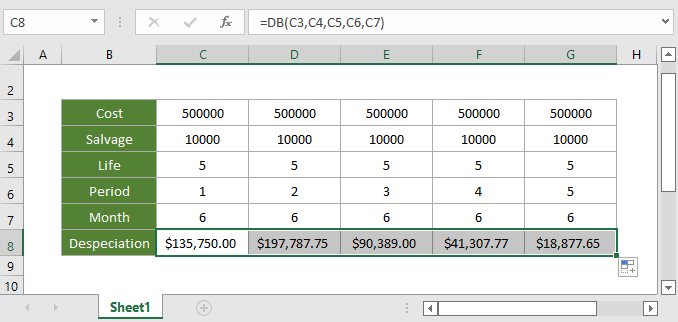In this case, in the first year, DB only depreciates over 6 months, and in the second year, DB depreciates over 18 months (6 months in year 1, and 12 months in year 2).

#### Relative Functions:

• Excel CUMIPMT Function
The CUMIPMT function returns the cumulative interest paid on a load between the start period and end period.

• Excel ACCRINT Function
The ACCRINT function returns the accrued interest on periodic interest-paying securities.

• Excel ACCRINTM Function
The ACCRINTM function returns the accrued interest for a security that pays interest at maturity.

• Excel AMORDEGRC Function
The AMORDEGRC function returns the linear depreciation of an asset for each accounting period by applying a depreciation coefficient based on the lifetime of the assets.

### The Best Office Productivity Tools

#### Kutools for Excel - Helps You To Stand Out From Crowd

 Popular Features: Find/Highlight/Identify Duplicates  |  Delete Blank Rows  |  Combine Columns or Cells without Losing Data  |  Round without Formula ... Super VLookup: Multiple Criteria  |  Multiple Value   |  Across Multi-Sheets  |  Fuzzy Lookup... Adv. Drop-down List: Easy Drop Down List  |  Dependent Drop Down List  |  Multi-select Drop Down List... Column Manager: Add a Specific Number of Columns  |  Move Columns  |  Unhide Columns  |  Compare Columns to Select Same & Different Cells ... Featured Features: Grid Focus  |  Design View  |  Big Formula Bar  |  Workbook & Sheet Manager  |  Resource Library (Auto Text)  |  Date Picker  |  Combine Worksheets  |  Encrypt/Decrypt Cells  |  Send Emails by List  |  Super Filter  |  Special Filter (filter bold/italic/strikethrough...) ... Top 15 Toolsets:  12 Text Tools (Add Text, Remove Characters ...)  |  50+ Chart Types (Gantt Chart ...)  |  40+ Practical Formulas (Calculate age based on birthday ...)  |  19 Insertion Tools (Insert QR Code, Insert Picture from Path ...)  |  12 Conversion Tools (Numbers to Words, Currency Conversion ...)  |  7 Merge & Split Tools (Advanced Combine Rows, Split Cells ...)  |  ... and more

Kutools for Excel Boasts Over 300 Features, Ensuring That What You Need is Just A Click Away...

Supports Office/Excel 2007-2021 and 365   |   Available in 44 Languages   |   Easy to Uninstall Completely#### Office Tab - Enable Tabbed Reading and Editing in Microsoft Office (include Excel)

• One second to switch between dozens of open documents!
• Reduce hundreds of mouse clicks for you every day, say goodbye to mouse hand.
• Increases your productivity by 50% when viewing and editing multiple documents.
• Brings Efficient Tabs to Office (include Excel), Just Like Chrome, Edge and Firefox.